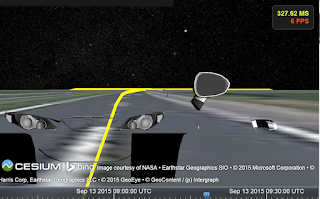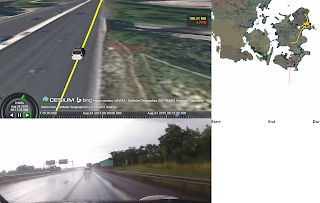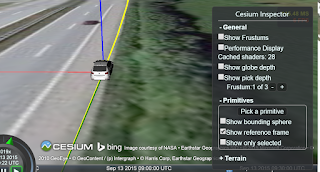# Finding heading from Position Property

I want to set the camera properties such it simulate looking out the front view of a car when driving and was thinking the best approach was to use the position property and sample two positions to then calculate the heading.

Its okay to find the math for doing this from two latlng pairs, but since I will be using either the camera lookAt or lookAtTransform (I have had a hard time figuring out in which cases one want to use the one or the other).

Is there anyone who can make a small example of using the Cesium API (Matrix,Transforms,Cartesian) to show how one would set the camera to be viewing out the front view, with both of above camera functions given two positions that illustrate two sampled position with dt difference.

var pos1 = new Cartesian();

var pos2 = new Cartesian();

LookAt, one would have to calculate the heading between the points.

LookAtTransform. Not sure how this is used.

Trying to understand in what cases the lookAtTransform is usefull.

Heres the code for the LookAt example:

``````  if (viewer.trackedEntity) {

var currentPos = viewer.trackedEntity.position.getValue(clock.currentTime);
if(oldPosition){
var pos1 = viewer.scene.globe.ellipsoid.cartesianToCartographic(oldPosition);
var pos2 = viewer.scene.globe.ellipsoid.cartesianToCartographic(currentPos);
var lat1 = pos1.latitude;
var lat2 = pos2.latitude ;
var lon1 = pos1.longitude ;
var lon2 = pos2.longitude ;
var tc1;

var dlat = lat2 - lat1;
var dlon = lon2 - lon1;
var y = Math.sin(lon2-lon1)*Math.cos(lat2);
var x = Math.cos(lat1)*Math.sin(lat2)-Math.sin(lat1)*Math.cos(lat2)*Math.cos(lon2-lon1);
if (y > 0){
if (x > 0) { tc1 = Math.atan(y/x);}
if (x < 0) { tc1 = Math.PI - Math.atan(-y/x);}
if (x === 0) { tc1 = Math.PI/2;}
}
if (y < 0) {
if (x > 0) { tc1 = -Math.atan(-y/x);}
if (x < 0) { tc1 = Math.atan(y/x)-Math.PI;}
if (x === 0) { tc1 = Math.PI/2*3;}
}
if (y === 0) {
if( x > 0) { tc1 = 0;}
if (x < 0) { tc1 = Math.PI;}
if (x === 0) {}
}

}
oldPosition = currentPos;
}
``````

});

``

Just took the first google hit so havent relfected over changing the code. this follows the entity 50 units behind .

I am wondering if there is something wrong with my heading calculation. As seen in the image, after setting camera inside the model.
setCarInsideView(scene: Cesium.Scene, pos: Cesium.Cartesian3, heading: number) {
var c = pos.clone();
c.z += 1;

``````}
``````

``

it looks alittle like the models orientation is abit off according to the path. (would expect the yellow path to go out in the middle between the two head lights.The heading calculation is as in previous post, and orientation of the model is set by.

``

also in the onTick callback

Are the some obvious mistake that I can have made?

Also when using a camera behind the model there seem to be some inconsitance about the heading and the path. Based on the code below I would expect the camera to behind the model and not like in the image here:setBehindCarView(scene: Cesium.Scene, pos: Cesium.Cartesian3, heading: number) {
var c = pos.clone();
c.z += 10;
scene.camera.setView.call(scene.camera, {
// position: c,
// roll : tc1 ,
});
}

``I believe this is related to some inconsistency with heading/pitch/roll that we were talking about in this post: https://groups.google.com/forum/?hl=en#!searchin/cesium-dev/i\$20have\$20an\$20update|sort:date/cesium-dev/uaQ2prOfwoY/r7rUr0MIDAAJ
I’m still waiting to hear an answer on that.
You are rotating the model to make it face forward, correct? I think the model is off center because of that.

For setBehindCarView, why are you adding 10 to c.z? I think that might be offsetting the camera incorrectly.

-Hannah

the position passed to the function is the cars current position and i wanted the camera to be lifted 10 meters and looking at a angle down the car from behind.

At this stage, due to not really having a background in computer graphics and not fully jeg understanding all the aspects of the lookat and setview of camera, the above code has been on a trial and error basis. So there is no real motivation behind why I did it like this. I am open to suggestions for better implementations.

Yes, i rotated the model to make it face the correct way according to driving location and when i used the build in VelocityOrientationProperty then the model was turned 90% degree according to driving location, so I could not use that. I guess it could take some additional parameters to flip instruct the property that the model is not using the correct axis.Just added a picture that also have the reference frame shown

Okay, I understand now. To accomplish this, I would recommend using camera.lookAtTransform. Here’s a demo:

var viewer = new Cesium.Viewer(‘cesiumContainer’, {
infoBox : false,
selectionIndicator : false
});

var url = ‘…/…/SampleData/models/CesiumGround/Cesium_Ground.bgltf’;
viewer.entities.removeAll();

var position = Cesium.Cartesian3.fromDegrees(-123.0744619, 44.0503706, 0);
var pitch = 0;
var roll = 0;

name : url,
position : position,
orientation : orientation,
model : {
uri : url,
minimumPixelSize : 128
}
});

var transform = Cesium.Matrix4.fromRotationTranslation(
Cesium.Matrix3.fromQuaternion(orientation, new Cesium.Matrix3()), position,
new Cesium.Matrix4());

viewer.camera.lookAtTransform(transform, new Cesium.Cartesian3(-100.0, 0.0, 10.0));

``

The transform puts the camera in the same reference frame as the model, which makes it easy to move the camera relative to the position of the model. You can use a Cartesian3 to define the offset. In this demo, x = -100 puts the camera 100m behind the model, and z=10 puts the camera 10m above the model.

For future reference, camera.lookAt/camera.lookAtTransform changes the camera transform. This locks the camera onto the target (which is the origin of the reference frame defined by the transform). We use this in the entity API to track entities. camera.setView moves the camera position and orientation but does not change the transform.

Hope this helps!

-Hannah

Thanks, it helps alot. I will play some more around with it and try your suggestion for moving the camera instead.